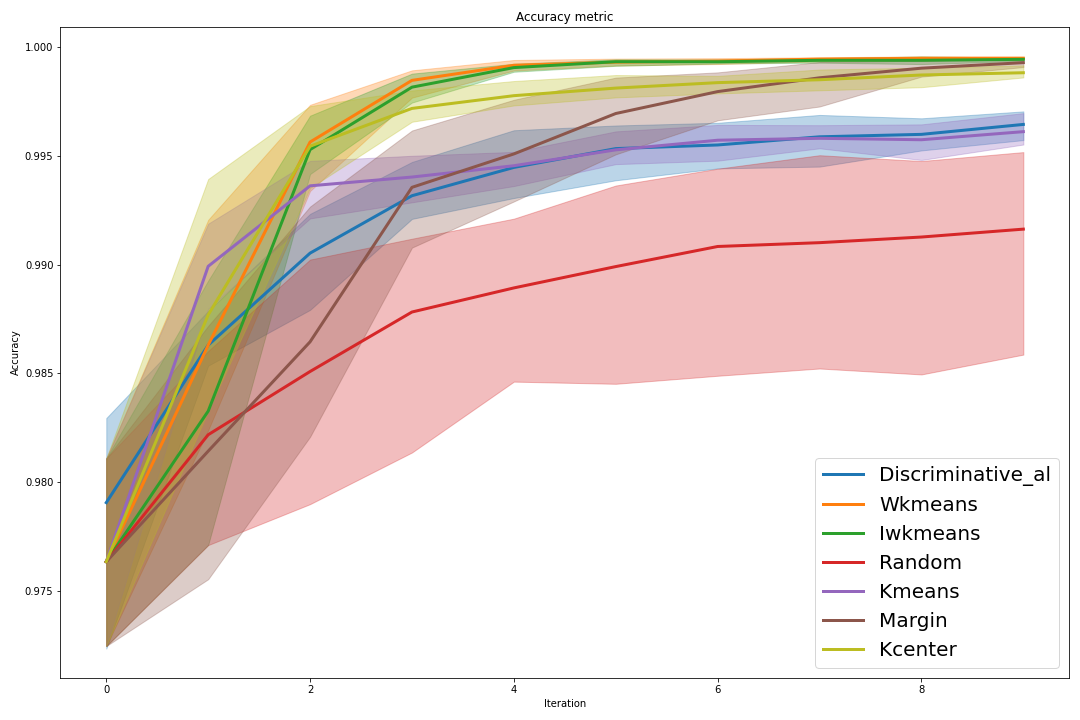# OpenAL: Evaluation and Interpretation of Active Learning Strategies

Tech Blog Alexandre Abraham

We are pleased to present our work accepted at the NeurIPS 2022 Workshop on Human in the Loop Learning! For a complete overview of our work, we refer to our paper and the OpenAL GitHub repository.

Despite being proven useful in practice, active learning (AL) suffers from two main drawbacks:

• Comparing results from different studies is complex. The literature is vast, and the validations are inconsistent across papers. Some AL techniques can even perform differently on similar tasks depending on the model used.
• Consequently, selecting the best sampler for a given task is challenging. Most papers report only the accuracy, which makes it difficult to develop an intuition of how the different AL strategies behave.

To address those limitations, we introduce OpenAL, a flexible and open source framework to efficiently run and compare sampling AL strategies on a collection of realistic tasks. The proposed benchmark is augmented with interpretability metrics and statistical analysis methods to understand when and why some samplers outperform others. Last but not least, practitioners can easily extend the benchmark by submitting their own AL samplers.

## The Simplest API

An AL experiment can be decomposed into three successive steps:

• Create initial conditions. Split the dataset between train and test for all folds and pick the initial labeled samples.
• Create experimental conditions. Pick the number of AL iterations and the batch size.
• Run the benchmark for various methods.

Running each of these steps is a simple one-liner in OpenAL. For example, if a practitioner would like to change the batch size of an experiment but keep the same initial conditions, it can be done very quickly.

`ic = load_initial_conditions(dataset_id)`
`ep = load_experiment(dataset_id, ic)`
`run(ep, methods)`

This blog post is complementary to the paper and describes how to run a task of a benchmark using a sampler for another package! The notebook corresponding to this tutorial is available here.

With similar goals but focused on images, Baidu research has recently published at NeurIPS, an extension of their DeepAL framework along with a comprehensive benchmark.

#### The Sampler Interface

To add a sampler, it must first comply with the internal interface used by OpenAL. We use the interface as defined by cardinal, which means that the sampler must have a fit method that takes as input labeled data and a select_samples method that takes as input the unlabeled ones. For this example, we use the DiscriminativeAL sampler:

`class WrappedDAL(BaseQuerySampler):    def __init__(self, batch_size: int, classifier):        super().__init__(batch_size)        self.classifier = classifier    def fit(self, X: np.ndarray, y: np.ndarray = None):        self.X, self.y = X, y        return self    def select_samples(self, X: np.array) -> np.array:        X_ = np.vstack([X, self.X])        y_ = np.hstack([np.full(X.shape, MISSING_LABEL), self.y])        qs = DiscriminativeAL(greedy_selection=True)        return qs.query(X=X_, y=y_, discriminator=self.classifier,                        batch_size=self.batch_size)`

Then, we simply load the initial conditions and experimental parameters of the benchmark for dataset 1502:

`ic = load_initial_conditions(‘1502’)ep = load_experiment(‘1502’, ic)gen_method = lambda params: WrappedDAL(    params[‘batch_size’], SklearnClassifier(params[‘clf’]))run(experimental_parameters, {‘DAL’: gen_method})`

## Results

OpenAL saves the results automatically as CSV files and updates the original plots. We can visualize them by looking at the graphs of the original experiment!

`acc_plt = ’experiment_results/1502/plots/plot-Accuracy.png’`
`display(Image(filename=acc_plt))`

We see that the discriminative sampler is better than random, close to K-Means in terms of performance. It is coherent with the fact that both samplers do not rely on model uncertainty. One thing to note is that we use the greedy version of the DiscriminativeAL that trains one classifier to select the whole batch. The non-greedy version is more performant but trains one model after selecting each sample which is prohibitive (the batch size being 245 on this dataset). A good compromise could be to retrain the model every 25 samples to perform only 10 trainings!